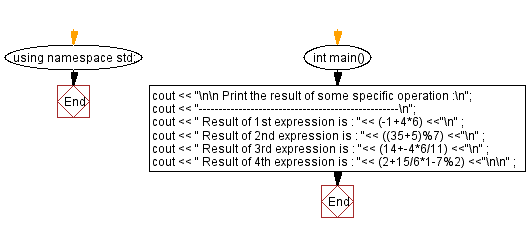﻿ C++ Exercises: Print the result of the specified operations - w3resource

# C++ Exercises: Print the result of the specified operations

## C++ Basic: Exercise-11 with Solution

Write a C++ program to print the results of the specified operations.

Sample Solution:

C++ Code :

``````#include <iostream>
using namespace std;

int main()
{
cout << "\n\n Print the result of some specific operation :\n";
cout << "--------------------------------------------------\n";
cout << " Result of 1st expression is : "<< (-1+4*6) <<"\n" ; //-1 + 24 = 23
cout << " Result of 2nd expression is : "<< ((35+5)%7) <<"\n" ; //40 % 7 = 5 (remainder of 40/7)
cout << " Result of 3rd expression is : "<< (14+-4*6/11) <<"\n" ;  //14 - (24/11)= 14 - 2 = 12
cout << " Result of 4th expression is : "<< (2+15/6*1-7%2) <<"\n\n" ; //2 + (15/6) - remainder of (7/2) = 2 + 2 - 1 = 4 - 1 = 3

}
``````

Sample Output:

``` Print the result of some specific operation :
--------------------------------------------------
Result of 1st expression is : 23
Result of 2nd expression is : 5
Result of 3rd expression is : 12
Result of 4th expression is : 3
```

Flowchart:C++ Code Editor:

What is the difficulty level of this exercise?

﻿

## C++ Programming: Tips of the Day

Why is there no std::stou?

The most pat answer would be that the C library has no corresponding "strtou", and the C++11 string functions are all just thinly veiled wrappers around the C library functions: The std::sto* functions mirror strto*, and the std::to_string functions use sprintf.

Ref: https://bit.ly/3wtz2qA

We are closing our Disqus commenting system for some maintenanace issues. You may write to us at reach[at]yahoo[dot]com or visit us at Facebook